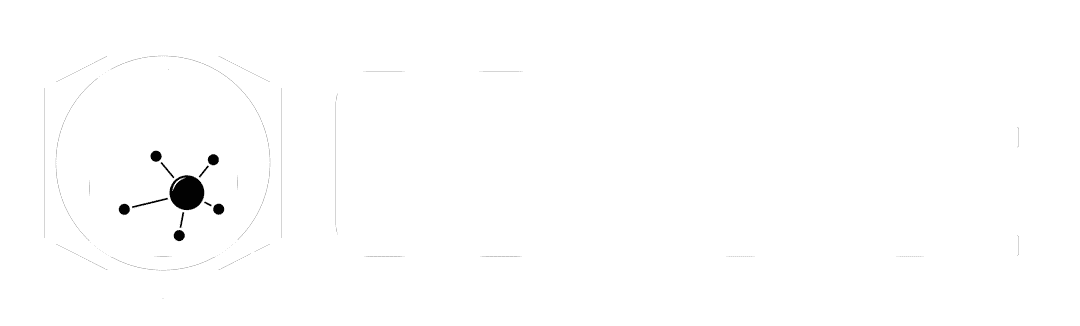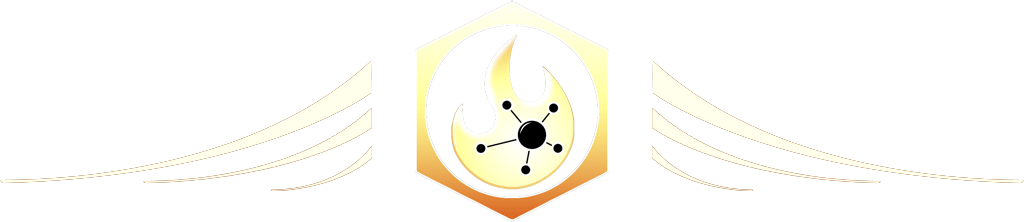# Sweep

The Ultimate Houdini node reference

# Visit the Node Bible

### Sweep

The Sweep SOP will convert a curve into geometry by creating either a tube, square tube, or wire with a custom profile.
Drag to resize

Summary:

The Sweep SOP is the node you want to use when converting curves into geometry. It has two inputs - the first is asking for the curve that you’d like to work on. The second is an optional input that will accept a “cross section” curve. Note that any geometry you plug into the second input must be a curve. If you try placing polygons into the second input, then it will create more geometry than necessary. Keep in mind that the @pscale attribute will be recognized by the sweep node, and this can control how thick the resulting geometry is along areas of the backbone curve.

In practice, this node is great for a wide variety of modeling tasks, hair/grooming tasks, and may also be useful for abstract FX work and text/logo reveals as well.

Drag to resize

General Parameters:

Backbone + Cross Section Curve Group:

-- If you’d like to specify a group within the first input, then you can do so here with the backbone curve group. For more info on groups, please visit the Group Node Bible entry.

Surface

Surface Shape:

-- Second Input Cross Sections = This is asking you for a shape to be specified within the second input of the node. This shape must be described by using a curve. It cannot be a polygon. It must face towards the +Z direction. Any curve can be accepted (NURBS, Bezier, Polygon, etc).
-- Round Tube = Instead of using the second input, it will create a round tube around the backbone.
-- Square Tube = Instead of using the second input, it will create a square tube around the backbone.
-- Ribbon = Instead of using the second input, it will create a flat grid at the location of the backbone.

Surface Type:

-- This section is asking you what kind of geometry you’d like to generate. By default, “Quadrilaterals” will make polygons with 4 sides. However, you can output a variety of other things like points, polylines, and triangle topology depending on your preference.  See below for visual examples: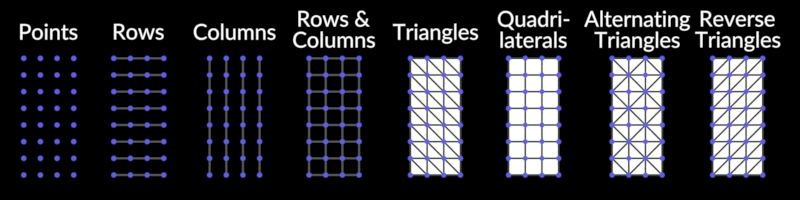Scale Cross Sections:

-- This allows you to scale the resulting geometry that’s generated by the sweep node.

Reverse Cross Sections:

-- If the resulting geometry is looking blue in your viewport, it’s because the normals are inverted. This will fix that issue by reversing the direction of the cross sections.

Stretch Around Turns:

-- When a curve makes a sharp change in direction, there’s a chance that the curve will lose some volume. This parameter compensates for this by “stretching” around the curves and adding a bit of extra volume to the curve.

Max Stretch:

-- This parameter controls the “Stretch Around Turns” function.

End Cap Type:

-- The end caps are the bits of geometry at the beginning and ends of the curve. Single polygon makes a single polygon without any divisions. Grid tries to make a quadrilateral grid out of the end cap. This mode also tries to make the end cap round (which can be controlled by the end cap roundness). The “Side Single Polygon” is a bit confusing, but according to the docs - “When the spine is closed but the cross-sections are open, the sides of the generated surface are open rather then the ends. This closes the sides with an N-gon.” Last but not least, you can group the end caps by turning on the “End Caps Group” parameter.

Apply Scale Along Curve:

-- This section represents the scale of the geometry from the beginning of the curve (left-hand size of the ramp) to the end of the geometry.

Rotate Order:

-- This section allows you to twist the resulting geometry as it goes from the beginning of the curve towards the end. In practice, it’s best to adjust the rotate order and turn up the “roll” parameter to see what it does. Likewise, you can just turn on the “Apply Yaw” and “Apply Pitch” to see what they do and if it makes a shape that you want. The topic of Pitch, Yaw, and Roll can become a lengthy explanation if you’re trying to technically understand it, however, in practice, it’s much easier to adjust these parameters until you have something that your eyes like.

Construction:

Cross Section Order:

-- The Sweep node can accept multiple curves in both the backbone and cross section inputs. According to the docs, “If there are multiple curves in the first (spine) input, the cross-section(s) is/are swept along each spine curve separately,” and “If there are multiple curves in the second (cross-section) input, the cross-sections are distributed along the curve length according to the Cross section order parameter on the Construction tab.” So... this means that you can place two curves into the second input to alternate between various shapes as the curve (or multiple curves) are being drawn out. These parameters are assuming that you have multiple curves plugged into the second (and perhaps first) input.

--  In the docs, it also describes this parameter - the “Cross Section Order,” as, “How the node decides what cross section(s) to use at each point along the spine curve.” To see this in action, observe what happens when we place two curves (one a circle and the other a square) into the second input and set the mode to “Cycle Through Cross Section Primitives per Curve.”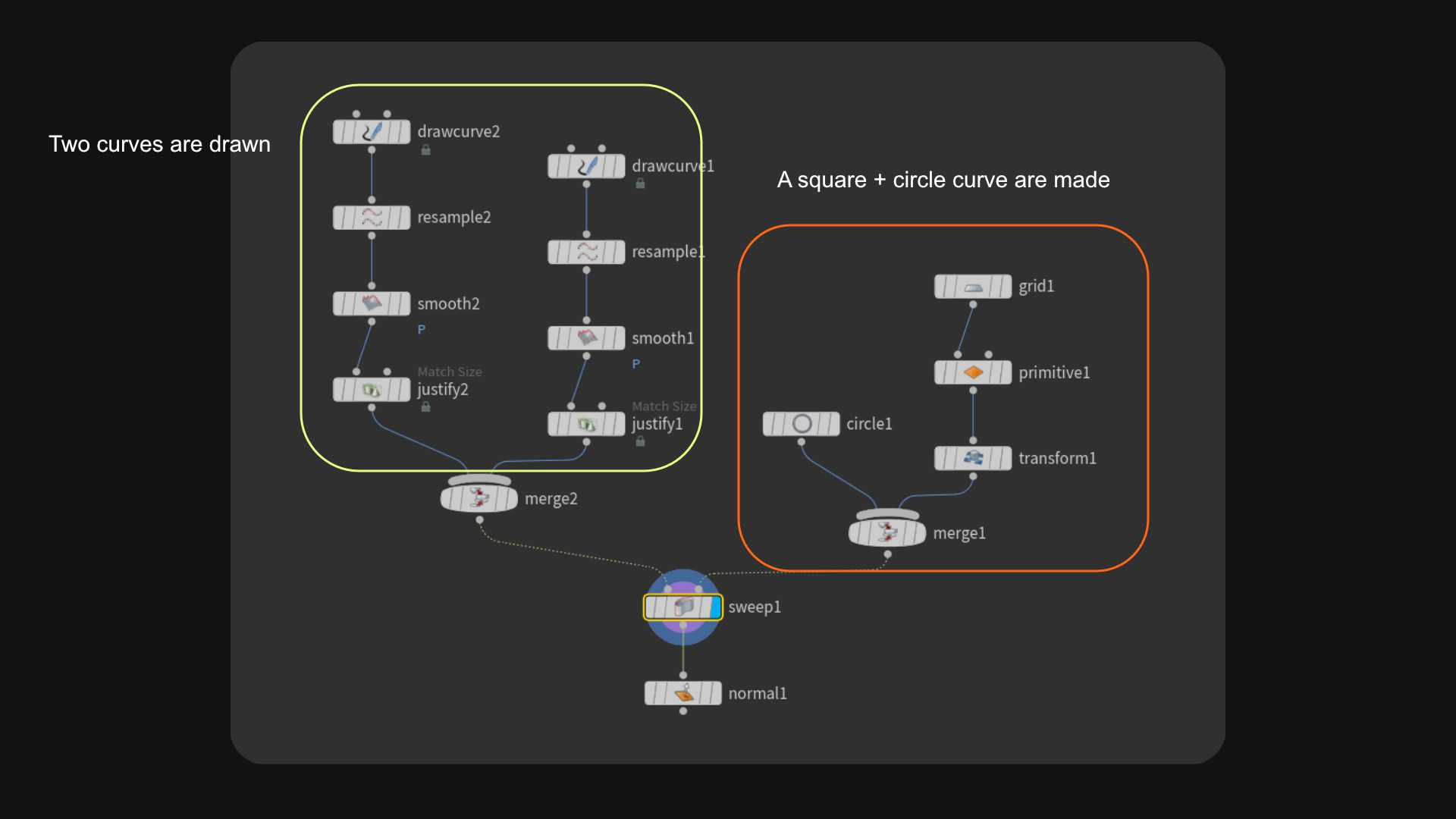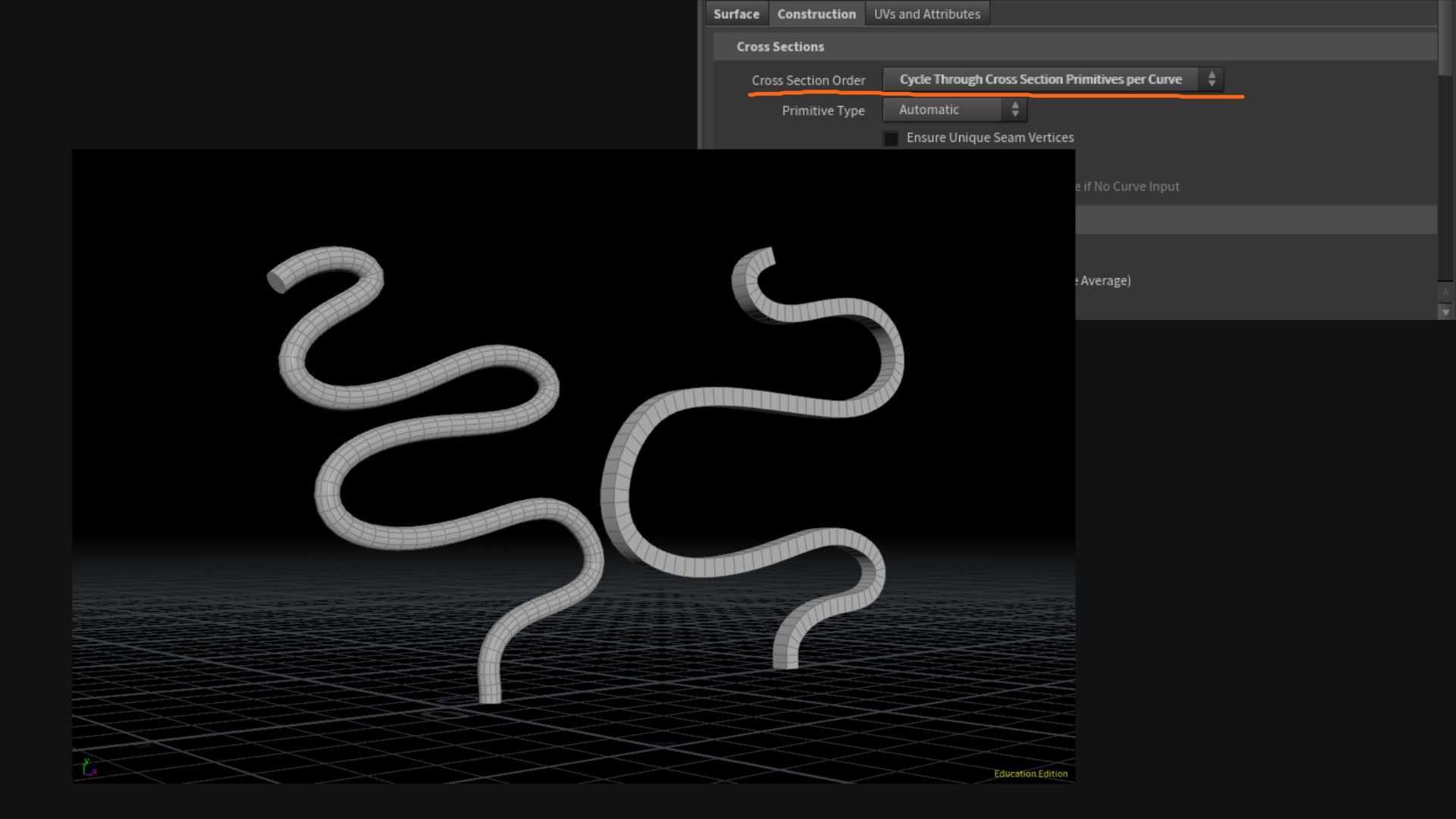--  As we can see, it has taken the two curves and assigned one to the circle cross section and the other to the square cross section.  In general, mixing and matching curves is what this tab is all about.  Now, when you read about the various options in the "Cross Section Order," it hopefully is more self-explanatory as to what it's doing.

Here is another mode as an example:

--  All Cross Sections At Each Curve Vertex: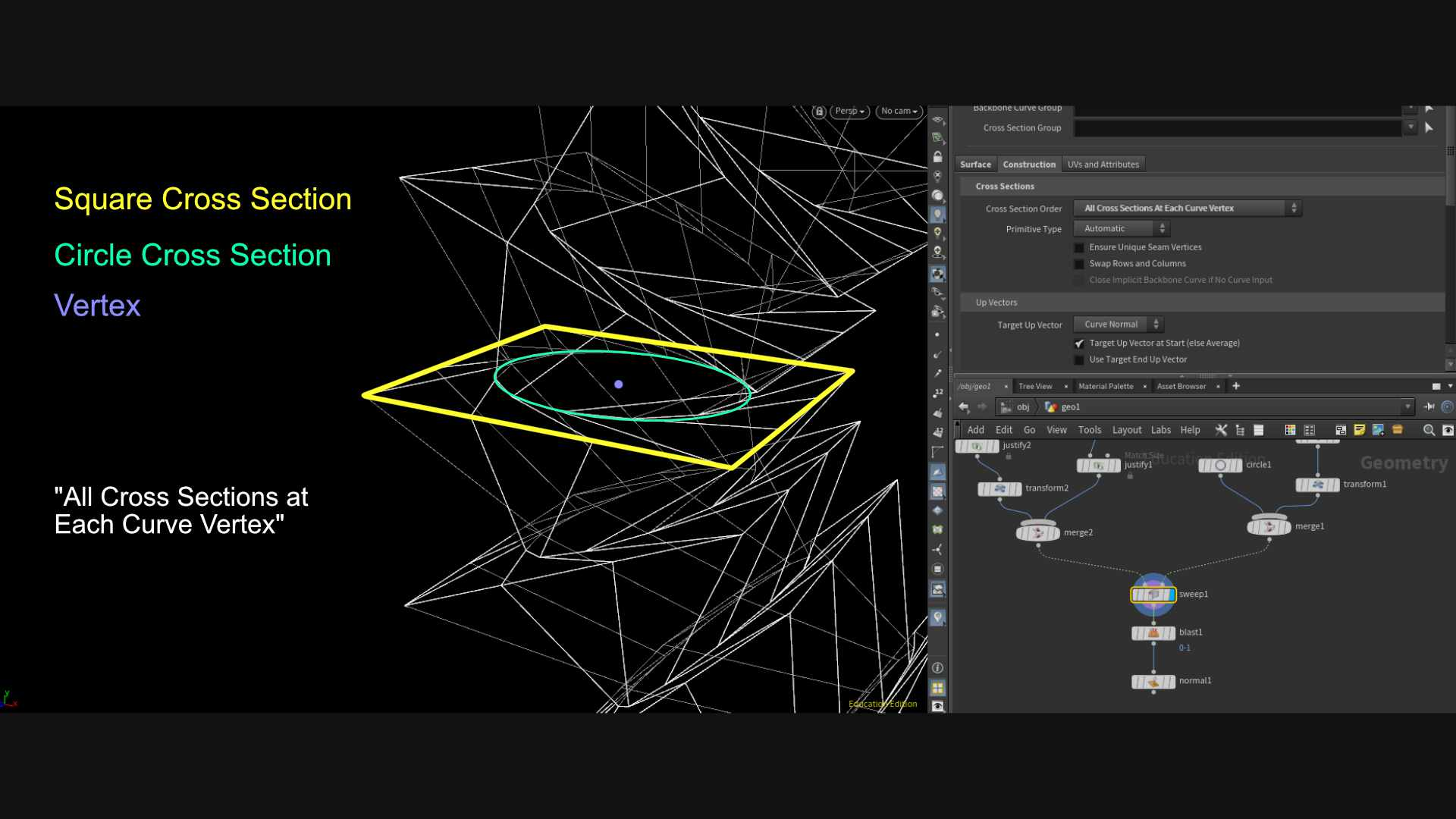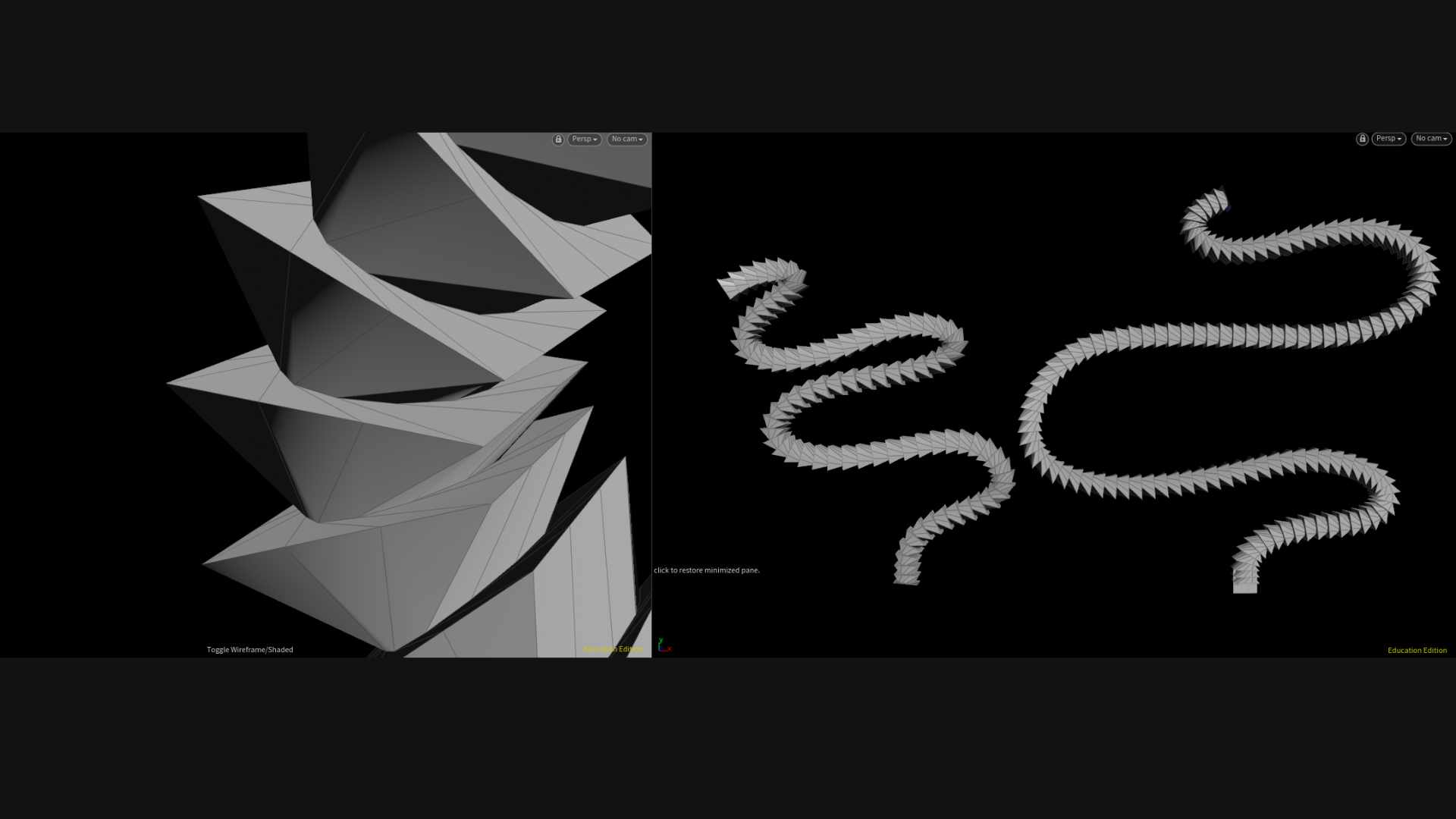The documentation also has examples which ought to make more sense now that you're familiar with what this section is trying to do.  The documentation is described as such below:

-- All Cross Sections at Each Curve Vertex =

Within a single curve’s output grid, at every single vertex, all cross sections will be used.

This is useful for if the cross sections collectively form a unit that should appear at each vertex within each grid, for example, to create a bulge at each vertex.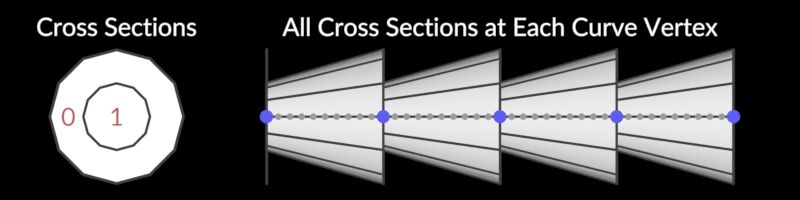-- Each Cross Section At All Curve Vertices =

Every curve will be separately surfaced once for each cross section.
This is useful if the cross sections should effectively act like a single, disconnected cross section, for example, to create multiple tubes for each curve.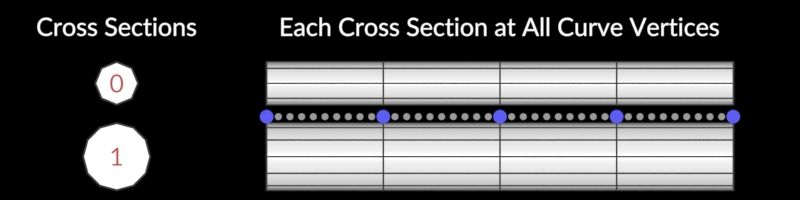-- Cycle Through Cross Section Primitives per Vertex =

Within each single curve’s output grid, the cross section selected for each vertex will cycle through the cross sections, starting from the first cross section.
This is useful if the cross sections form all cross sections to be used along the curves in order, for example, if the cross section input contains all cross sections for a corridor in order.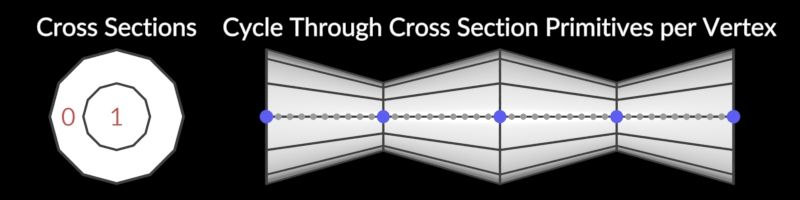-- Cycle Through Cross Section Primitives per Primitive =

Within each single curve’s output grid, the cross section selected for each vertex will cycle through the cross sections, starting from the first cross section.
This is useful if the cross sections form all cross sections to be used along the curves in order, for example, if the cross section input contains all cross sections for a corridor in order.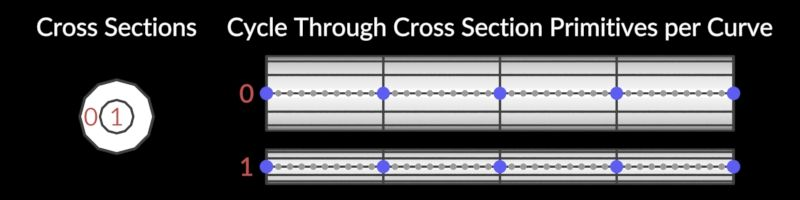-- Choose Cross Section Primitives by Attribute =

For each vertex, select a cross section to use based on the attribute specified in the Cross Section Attribute parameter.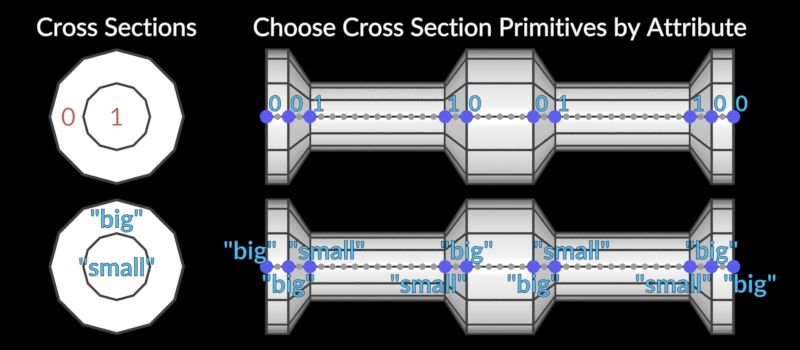Moving on now...

Primitive Type:

-- This is asking you what kind of geometry you'd like to make. If you'd like to make NURBs surfaces, polygons, or other types of primitives, this is where you can specify so. Automatic will take a look at the primitive type of the incoming backbone curve(s) and determine what sort of primitive type the resulting geo should be based on that.

Ensure Unique Seam Vertices:

-- According to the docs, “If Surface Type is “Rows”, “Columns”, or “Rows and Columns”, and the spine curve or cross-section is closed, this adds an extra vertex (if needed) to each curve to ensure that there are separate vertices for parametric curve u or v coordinate values 0 and 1.
If any output primitives are polygon soups, NURBS surfaces, Bezier surfaces, or bilinear mesh primitives, this option similarly also adds any extra vertices that would be needed to ensure that all uv seams are parameterized correctly.”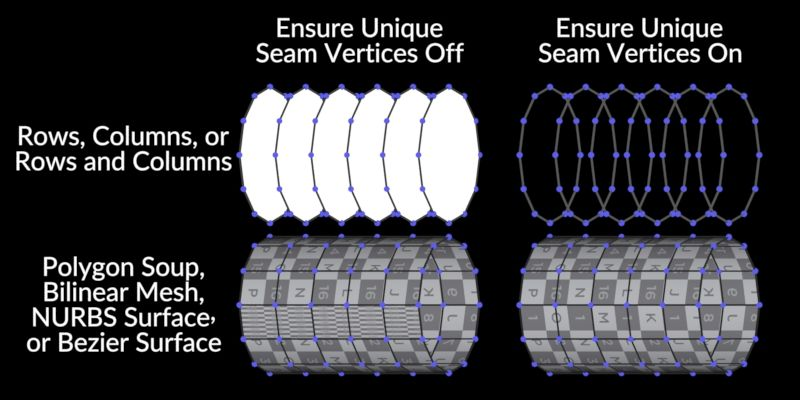-- In practice, you probably won’t need to adjust this parameter unless you are trying to create curves because it’s only going to matter when you’re working with NURBS, Bilinear Meshes, Polygon Soups, or Bezier Surfaces. Most of the time when you work with those other surfaces, you’ll end up converting back to polygons anyway, so it’s not something you run into often.

-- Also, by default, Houdini will not close the curves that are created by the rows and columns options. In order to create closed curves, you must turn this parameter on.

Swap Rows and Columns:

-- In practice, this parameter may be useful when trying to invert your normals.

Close Implicit Backbone Curve if No Curve Input:

-- This parameter is confusing and appears to not function correctly. In this workflow, it is assumed that you are not placing a backbone curve into the first input. If you do this, the node will error out and tell you that you need to place something in the first input. So, I’m not sure what the intention is for this setting, and it’s probably best to avoid it.

Target Up Vector + Tangents:

-- When the sweep node is trying to generate geometry, it basically copies shapes onto the points along the curve, and then does something to those shapes to generate the geometry. In other words, it basically does this sort of thing: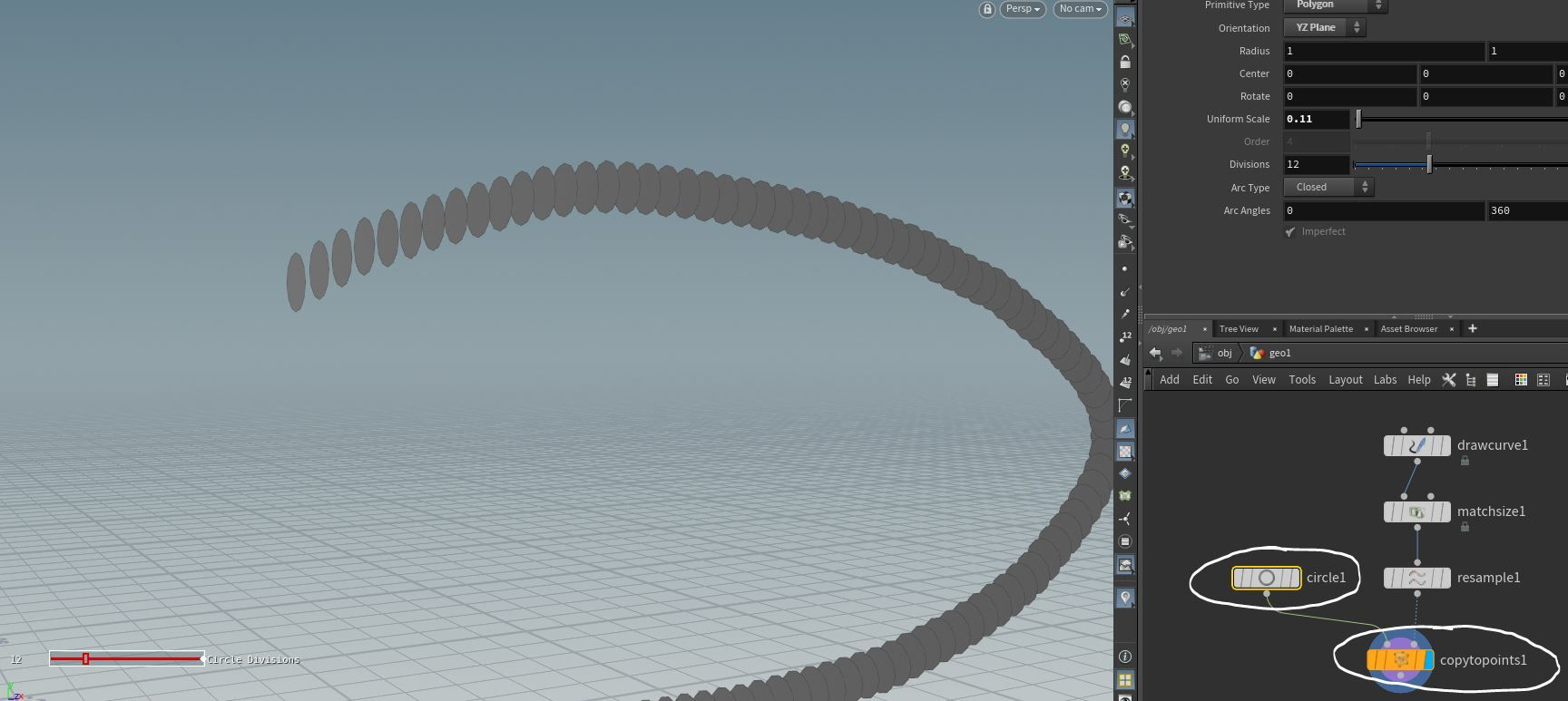-- And then, once it has those bits of geometry along the curve, it figures out what needs to happen in order to give you the geometry you're looking for.  Well... in the process of copying those shapes over to the points, there needs to be a way of properly orienting those shapes, and the next two sections deal with how the points and geometry are oriented.

-- The “up” vector will only affect the shape if you are using a curve from the second input because it is trying to describe which way is “up” for the curve in the second input. In practice, I've found this section to be a bit unpredictable, so it’s best to just orient your cross-section curve facing in the Z direction and not mess with the defaults.

--  The tangent type parameters are trying to determine the orientation of the backbone curve.  According to the documentation...

--  Average of Edge Directions = "Average of the normalized directions of the previous and next edge."

--  Central Difference = "Average of the non-normalized previous and next edge vectors.
This is similar to the “Central Difference” option for Velocity Approximation on the Trail node."

--  Previous Edge = "Previous edge direction."

--  Next Edge = "Next edge direction"

--  Z Axis (Ignore Curve) = "The tangent will be chosen as the Z axis, regardless of the curve.
This can be useful if the cross sections have already been rotated to the desired orientation."

The diagram in the docs does a much better job at illustrating these concepts: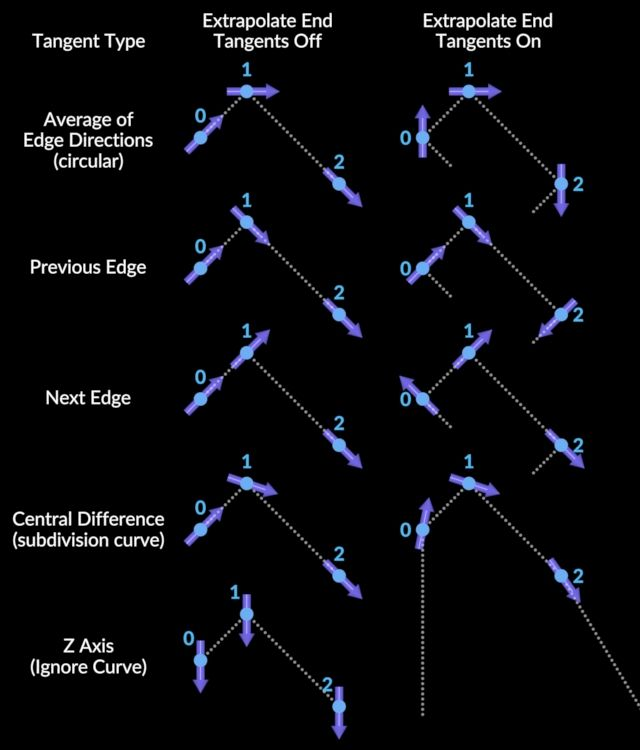Extrapolate End Tangents:

-- If you’re having issues with the orientation of the faces at the end of your curve, then this parameter may help. This rotates the end of the curve so that its orientation makes more sense based on the direction that the rest of the curve has been going.

-- In other words, if you’re determining the direction based on edges that are in front of a vertex (Which is what happens when the Tangent Type parameter is set to average or central difference) then there is no vertex ahead of the last vertex of your curve, and that’s a problem.  So, to make sure that the direction is consistent with everything else, the sweep node will make another vertex ahead of the last one and figure out the orientation based on that “Extrapolated” position. Hence the name of the parameter, “Extrapolate End Tangents.”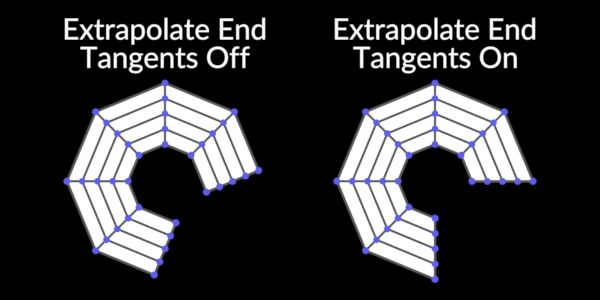Transform Using Curve Point Attributes:

-- According to the docs, "You can also scale the cross-section using pscale or scale point attributes on the spine curve, and orient the cross-sections using the point attributes P, N, up, orient, rot, trans, pivot, and/or transform on the spine curve.  If N is present and none of up, orient, rot, or transform are present, the node uses N as the curve tangent and computes the rest of the rotation as usual.  If up is present and none of N, orient, rot, or transform are present, the node computes the tangent as usual, and uses up as the up-vector at each vertex (before any roll or twist is applied)."

UVs and Attributes:

-- In most situations, you will not need to adjust the default settings for the uvs. Just in case you do what to change those defaults, however, there’s a ton of options to do so here.  This section also contains various options for attributes to export.  So, if you're looking for an attribute that describes something, there's a good chance you'll find it here.

Compute UVS:

-- “Generate a uv vertex attribute on the generated surface. If the input curves already have uv attributes, the node uses the values from those attributes (U corresponds to the cross sections, and V corresponds to the spine curve).  (If Surface Type is “Points”, this will generate a point attribute.)”

Override Any Existing UVs:

-- “When Compute UVs is on, compute new UVs for the surface, even if the input curves already had uv attributes.”

Length-Weighted UVs:

-- “Length-Weighted UVs
Scale the U and V coordinates based on proportional edge lengths so they're even across the whole surface, rather than bunching up where edges bunch up. (If Normalize Computed Us and/or Normalize Computed Vs are on, the coordinates are still normalized to be within the 0 to 1 range.)”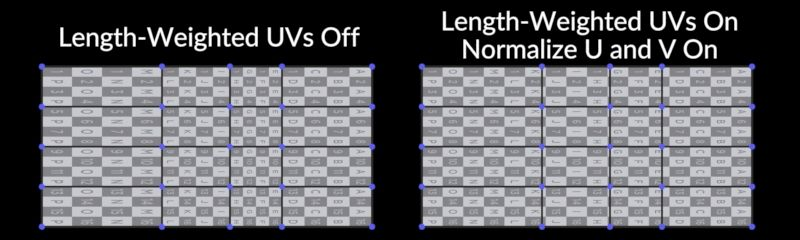Normalize Computed Us + Computed Vs:

-- These parameters will basically removes uv distortion if you turn on the “normalize computed Us” and fit everything neatly into one uv square if you turn on “Normalize Computed Vs.” It can be helpful when you want a repeating texture and/or prevent distortions.

-- Here's the complex explanation:  According to the docs, “Normalize computed U coordinates to be in the range 0 to 1 (before applying UV scale). When this is off, U corresponds to the average distance around the cross sections on the mesh (ignoring any stretch applied by Stretch Around Turns). When this is on and Normalize Computed Vs is off, the node scales V such that U and V appear scaled similarly in space (depending on the Use Max Cross Section Length per Curve for Proportional Scale setting). This is especially useful for things like applying repeating rope textures, where the texture should be applied isotropically.”

-- And similarly for the Normalize Computed V.... “Normalize computed V coordinates to be in the range 0 to 1 (before applying UV scale). When this is off, V corresponds to the average distance along the surface in V, or the distance along the spine curve, depending on the Use Mesh Edge Lengths instead of Curve Edge Lengths parameter. When this is on and Normalize Computed Us is off, the node scales U such that U and V appear scaled similarly in space.”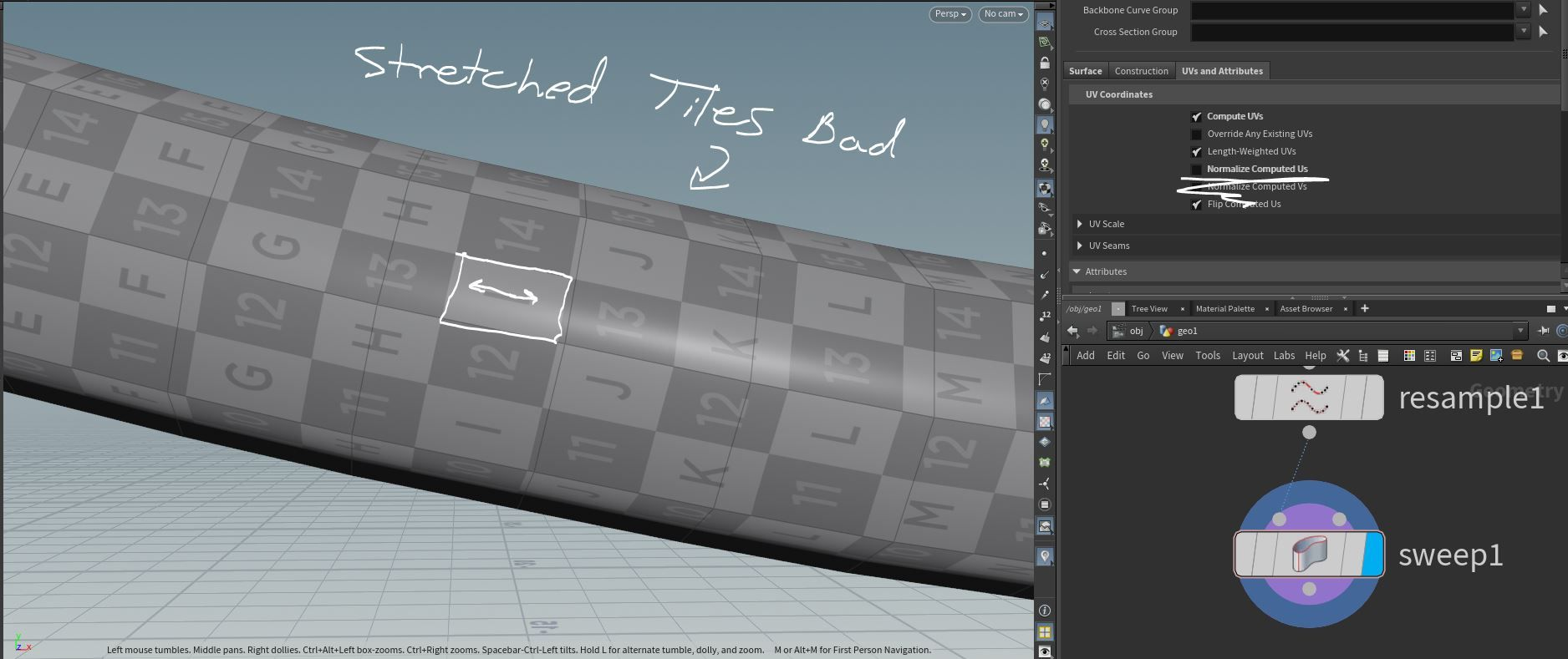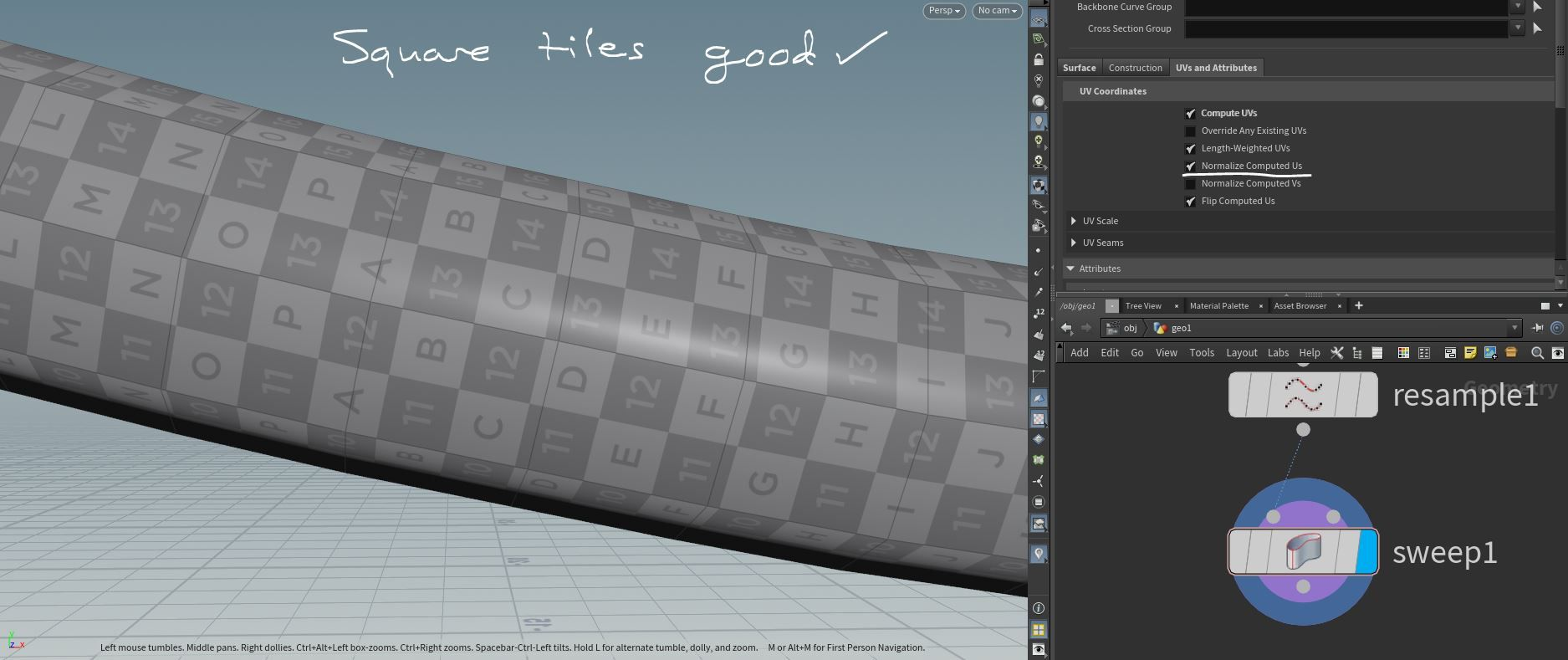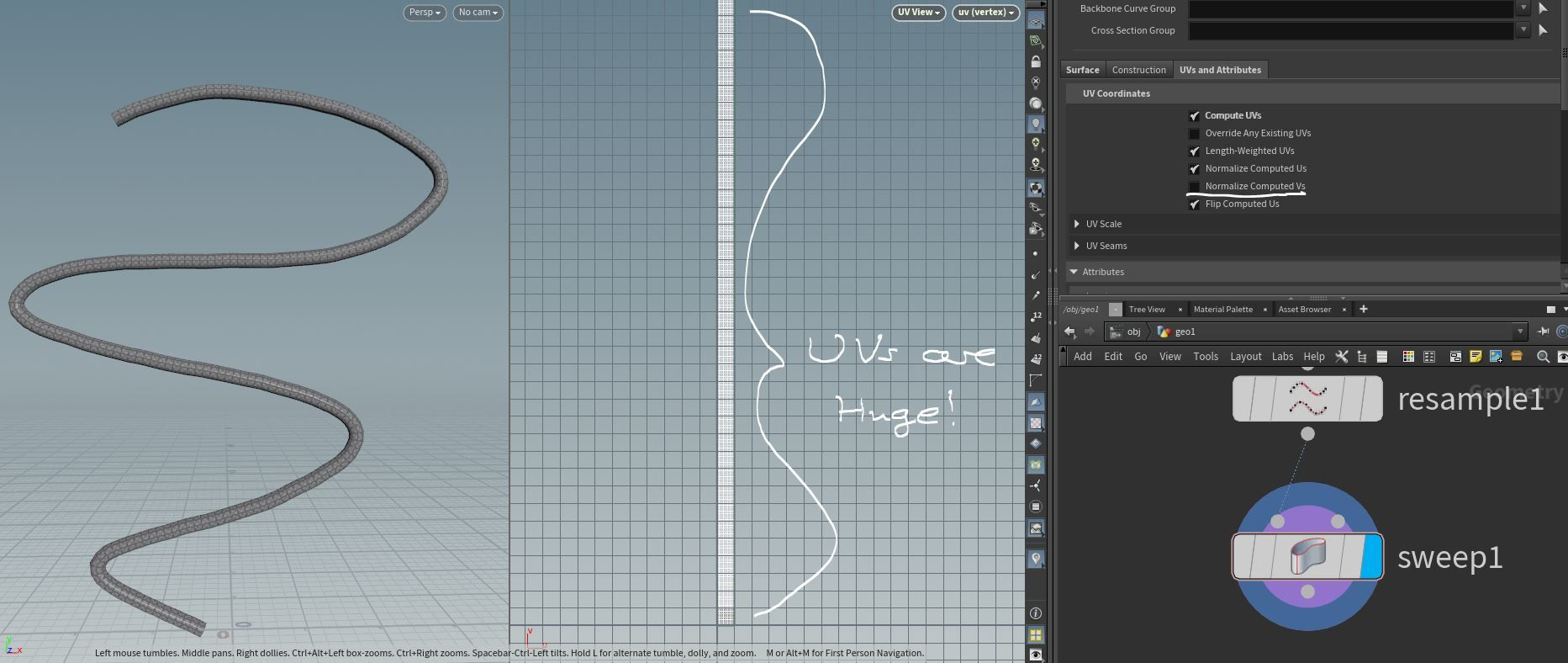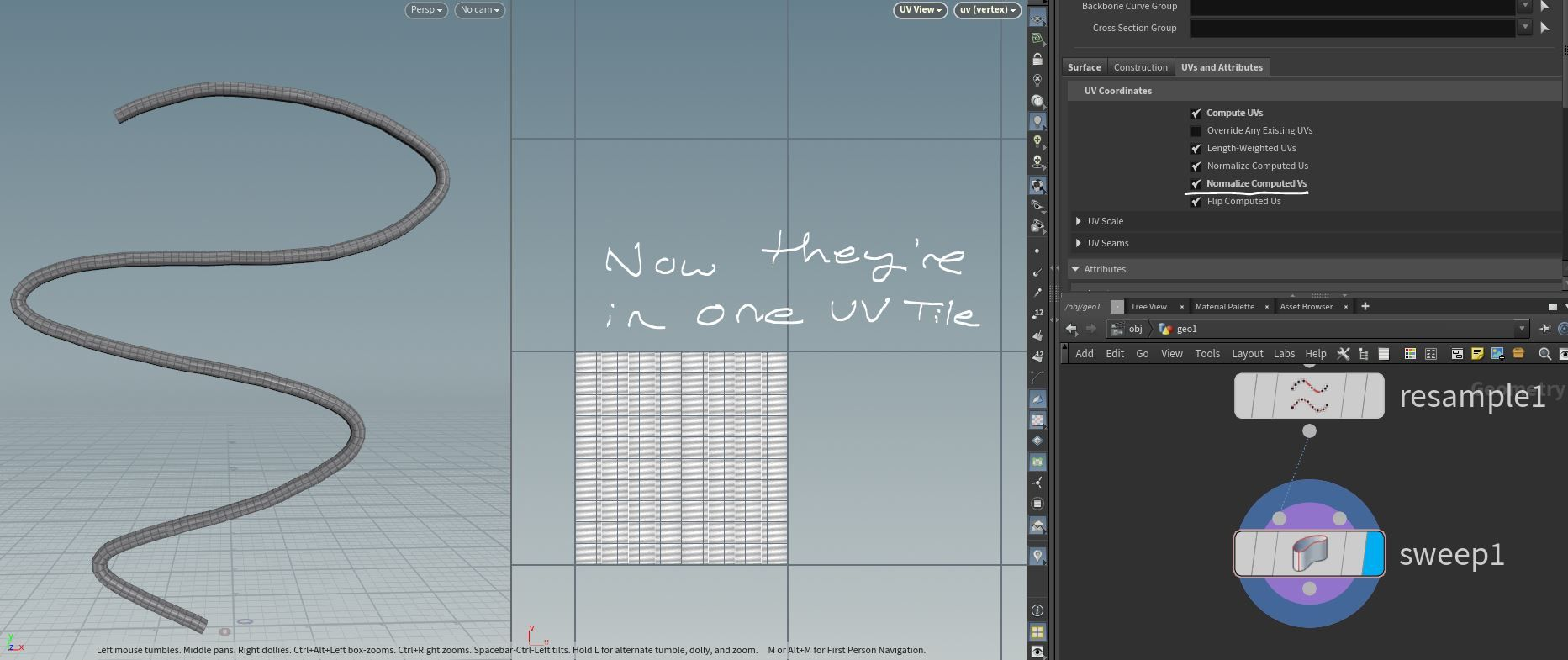Flip Computed Us:

-- If you notice that the letters are backwards on your UV tiles, then it's probably because your normals are reversed, so you should fix that.  Otherwise, you can also use this parameter to flip the uvs in the U direction.

UV Scale:

-- Change this parameter to scale the uvs.  Keep a split screen like what I have in the screenshot above and see what happens.

Use Mesh Edge Lengths Instead of Curve Edge Lengths + Max Cross Section Length per Curve for Proportional Scale:

--  In practice, I have never found a practical use for these settings, so I would suggest avoiding them.

Snap U + V to the Nearest Tile Boundary:

--  This will do as it says in the name.

From Backbone Curves:

--  This area will control which attributes are present in the final mesh.  The attributes here are derived from the first input.

From Cross Sections:

-- This area will control which attributes are present in the final mesh. The attributes here are derived from the second input.

--  In general, I would suggest experimenting with these attributes if you have a specific situation that requires them.  I'm not going to cover what an applicable scenario would be for each attribute, but just know that it's all here if you need it for a specific situation.

Drag to resize